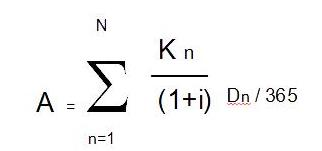• Months
Maximum period
• AMD
Maximum laon amount
• People
Got a loan

Real interest rate's calculation code

Example of real interest rate’s calculation

The real interest rate is calculated by using the following formula:Where,

i = annual real interest rate

A = initial amount of the loan

n = the amount of customer’s payments (including loan payments, interest payments and other payments)

N = the number of last loan payment

Kn = shows the amount of the ordinary n-th payment (loan payment, interest payments and other payments) at the moment of loans assignment and during the loan’s duration

D= shows at the moment of loan assignment until the loan’s ordinary n-th payment including, nevertheless the day of loan assignment is not included in the calculation.

Examples

Example 1

-the amount of the loan – 1,500,000 AMD

- the loan repayment term– 24 months

-annual nominal rate – 22%

-the loan repayment mode – annuity

- other single payments done by the customer the day of loan assignment

• Application review fee – 10 000 AMD
• Commissions= 15 000 AMD

-other lump sum  payments related to loan assignment

-          lump sum of estate valuation  – 15 000 AMD

The annual real interest rate of the loan mentioned above is 27.94%.

Example 2

-the amount of the loan – 3,000,000 AMD

- the loan repayment term – 36 months

-annual nominal rate – 21%

-the loan repayment mode – annuity

- other lump sum payments done by the customer the day of loan assignment

• Application review fee – 10 000 AMD
• Commissions = 24 000 AMD

-other lump sum payments related to loan assignment

-          estate valuation – 15 000 AMD

The annual real interest rate of the loan mentioned above is 24.64%.

Example 3

-the amount of the loan – 6,000,000 AMD

- the loan repayment term – 48 months

-annual nominal rate – 19%

-the loan repayment mode – annuity

- other lump sum payments done by the customer the day of loan assignment

• Application review fee – 10 000 AMD
• Commissions= 42 000 AMD

-other lump sum  payments related to loan assignment

-          lump sum  of estate valuation – 20 000 AMD

The annual real interest rate of the loan mentioned above is 21.57%.

Example 4

-the amount of the loan – 8,000,000 AMD

- the loan repayment term – 54 months

-annual nominal rate – 18%

-the loan repayment mode – annuity

- other lump sum  payments done by the customer the day of loan assignment

• Application review fee – 10 000 AMD
• Commissions= 56 000 AMD

-other lump sum  payments related to loan assignment

-          lump sum   of estate valuation – 30 000 AMD

The annual real interest rate of the loan mentioned above is 20.29%.

How to get a loan
• Application
• Preparation of documents
• Loan confirmation
LOAN BENEFITS